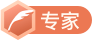# LeetCode 30串联所有单词的子串&31下一个排列

## 串联所有单词得字串

s = “barfoothefoobarman”,
words = [“foo”,“bar”]

s = “wordgoodgoodgoodbestword”,
words = [“word”,“good”,“best”,“word”]• words中所有单词长度都相同
• 必须使用所有words中的单词一次public List<Integer> findSubstring(String s, String[] words)
{
List<Integer>value=new ArrayList<Integer>();
Map<String, Integer>map=new HashMap<String, Integer>();
for(int i=0;i<words.length;i++)
{
int num=map.getOrDefault(words[i], 0);
map.put(words[i], num+1);
}
int wordlen=words.length();
int len=words.length()*words.length;
StringBuilder sBuilder=new StringBuilder(" ");
sBuilder.append(s.substring(0, len-1));
for(int i=0;i<s.length()-len+1;i++)
{
sBuilder.deleteCharAt(0);
sBuilder.append(s.charAt(i+len-1));

int num=0;//统计总共满足的单词数量
Map<String, Integer>map2=new HashMap<String, Integer>();
//map2.putAll(map);
int index=0;
while (index<len) {
String team=sBuilder.substring(index,index+wordlen);
int number=map2.getOrDefault(team, 0);//次数
map2.put(team, number+1);
if(number+1>map.getOrDefault(team, 0))
break;

index+=wordlen;
}

if(index==len)

}
return value;
}Hash滑动窗口优化• 遇到新单词不存在，此时j和index都移动到单词后重新开始，且储存的动态map需要clear；• 遇到的单词存在，但是多了，需要将j右移一直到消除这个多余单词，同时修改动态map。• 正常情况，叠加匹配更新index和map。public List<Integer> findSubstring(String s, String[] words) {
List<Integer>value=new ArrayList<Integer>();//返回的结果
Map<String, Integer>map=new HashMap<String, Integer>();//统计单词个数
for(String team:words)//进行统计
{
int num=map.getOrDefault(team, 0);
map.put(team, num+1);
}
int wordlen=words.length();//单个单词的长度
int len=words.length()*words.length;//总长度
for(int i=0;i<wordlen;i++)//分组分别进行
{
int j=i,index=j;
Map<String, Integer>map2=new HashMap<String, Integer>();
while (j<=s.length()-len&&index+wordlen<=s.length()) {
String word=s.substring(index,index+wordlen);

int num=map2.getOrDefault(word, 0);
map2.put(word, num+1);
if(!map.containsKey(word))//不包含该元素，直接跳过
{

j=index+wordlen;
map2.clear();
}
else if(map.get(word)<num+1)//元素存在但次数过多
{
String teamstr="";
while (!(teamstr=s.substring(j,j+wordlen)).equals(word)) {//找到第一个不相等得
map2.put(teamstr, map2.get(teamstr)-1);
j+=wordlen;
}
map2.put(teamstr, map2.get(teamstr)-1);
j+=wordlen;
}
index+=wordlen;
if(index==j+len)
{
while (index+wordlen<=s.length()&&s.substring(j, j+wordlen).equals(s.substring(index, index+wordlen))) {
j+=wordlen;index+=wordlen;
}
String teamstr=s.substring(j,j+wordlen);
map2.put(teamstr, map2.get(teamstr)-1);
j+=wordlen;
}

}
}
return value;
}## 下一个排列

1,2,3 → 1,3,2
3,2,1 → 1,2,3
1,1,5 → 1,5,1

• 例如1 2 3 5 4 交换3和4成为1 2 4 5 3.

• 例如上述变成1 2 4 3 5；

 public void nextPermutation(int[] nums) {
boolean jud=false;
int i,j=0;
for( i=nums.length-2;i>=0;i--)
{
for( j=nums.length-1;j>i;j--)
{

if(!jud&&nums[i]<nums[j])
{
int team=nums[i];
nums[i]=nums[j];
nums[j]=team;
jud=true;
break;
}
}
if(jud)break;
}
if(jud)
for(int k=nums.length-1;k>i;k--)
{
for(int m=k-1;m>i;m--)
{
if(nums[k]<nums[m])
{
int team=nums[k];
nums[k]=nums[m];
nums[m]=team;
}
}
}
int team;
if(!jud)
for( i=0;i<nums.length/2;i++)
{
team=nums[i];
nums[i]=nums[nums.length-1-i];
nums[nums.length-1-i]=team;
}
}Big saiCSDN认证博客专家 数据结构与算法 爬虫 Java

01-251150
10-2352901-27338
05-261341
01-272146
08-25255
09-12292
04-17330
04-221588
04-25189点击重新获取扫码支付余额充值